Latest Banking jobs   »

# Quantitative Aptitude Quiz For SBI Clerk Prelims 2022- 15th September

Directions (1-5): In each of these questions, two equations (I) and (II) are given. Solve the equations and mark the correct option:

Q1.
I: 2x² + 10x + 12 = 0
II: y² + 10x + 25 = 0
(a) x > y
(b) x ≥ y
(c) x < y
(d) x ≤ y
(e) x = y or relation can’t be established.

Q2.
I. x² – 5x + 6 = 0
II. y² + 7y + 6 = 0
(a) x > y
(b) x ≥ y
(c) x < y
(d) x ≤ y
(e) x = y or relation can’t be established.

Q3.
I. x² = 625
II. y = √625
(a) x > y
(b) x ≥ y
(c) x < y
(d) x ≤ y
(e) x = y or relation can’t be established.

Q4.
I. 2x – 3y = 0
II. 4x – 2y = 16
(a) x > y
(b) x ≥ y
(c) x < y
(d) x ≤ y
(e) x = y or relation can’t be established.

Q5.
I. x³ = 1331
II. y = ∛1331
(a) x > y
(b) x ≥ y
(c) x < y
(d) x ≤ y
(e) x = y or relation can’t be established

Directions (6-10):- In each of the following questions, two equations (I) and (II) are given. Solve the equations and mark the correct option:
(a) if x>y
(b) if x≥y
(c) if x<y
(d) if x ≤y
(e) if x = y or no relation can be established between x and y.

Q6.
I. x²+17x+72=0
II. y²+11y+30=0

Q7.
I. 3x²-23x+40=0
II. 5y²-17y+14=0

Q8.
I. x²-26x+168=0
II. y²-29y+208=0

Q9.
I. x³+340=2537
II.y²+23=192

Q10.
I. x²+48x+575=0
II. y²+44y+483=0

Directions (11-15):- In each of the following questions, two equations (I) and (II) are given. Solve the equations and mark the correct option:
(a) if x>y
(b) if x≥y
(c) if x<y
(d) if x ≤y
(e) if x = y or no relation can be established between x and y.

Q11. I. x²+23x+132=0
II. y²+21y+110=0

Q12. I. 3x²+20x+32=0
II. 5y²+23y+24=0

Q13. I. x²-29x+208=0
II. y²-21y+108=0

Q14. I. x²+30x+224=0
II.y²+35y+306=0

Q15. I. x= ∛4096
II. y² =256

Solutions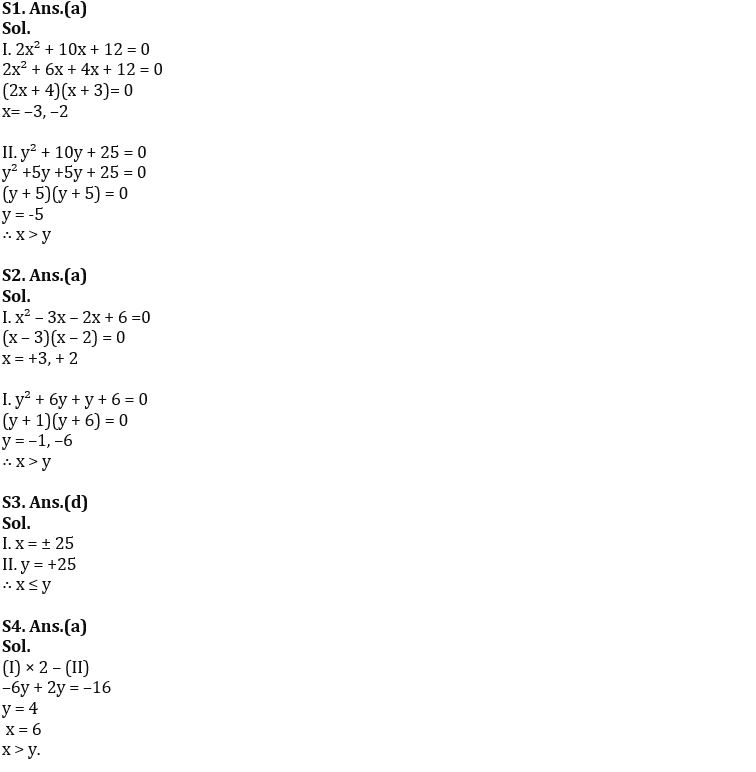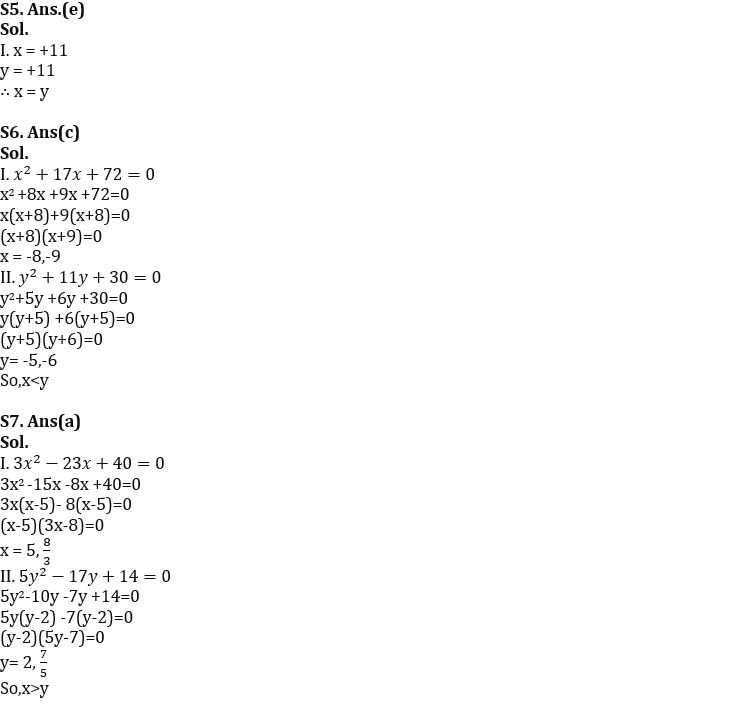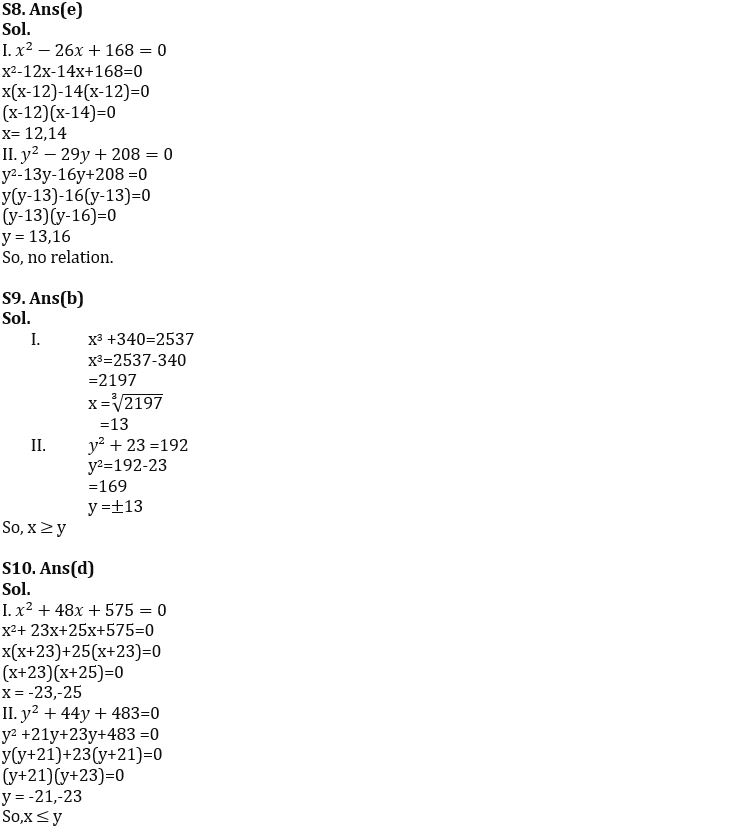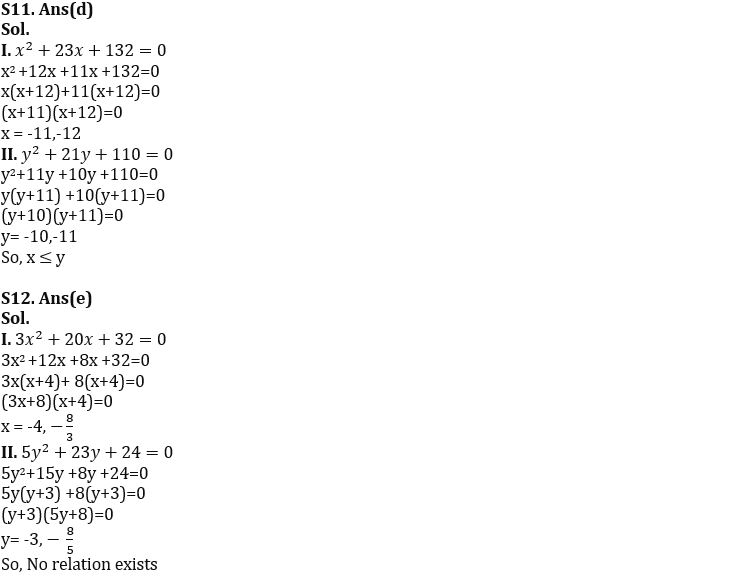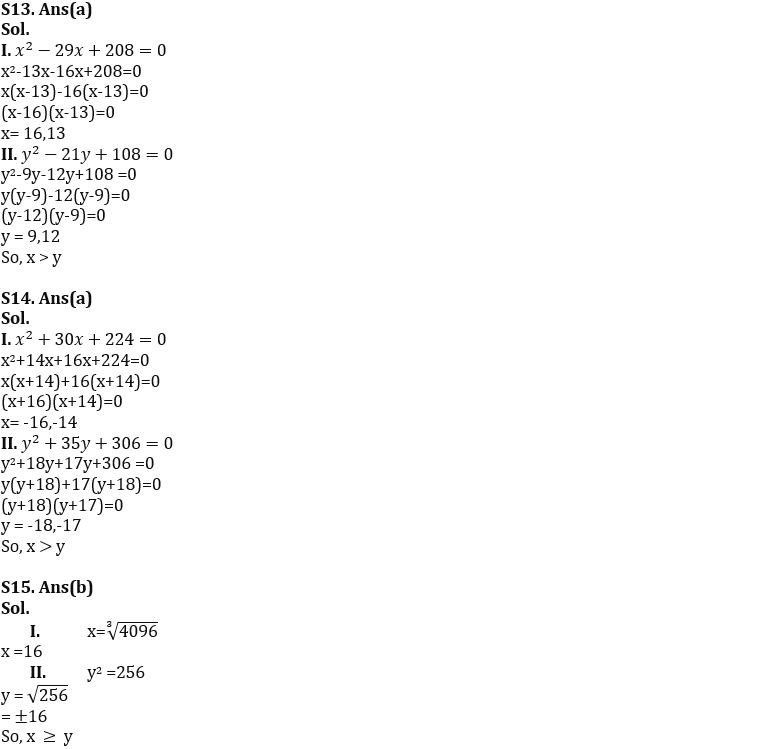#### Congratulations!Download Hindu Review of October 2021: Free PDF